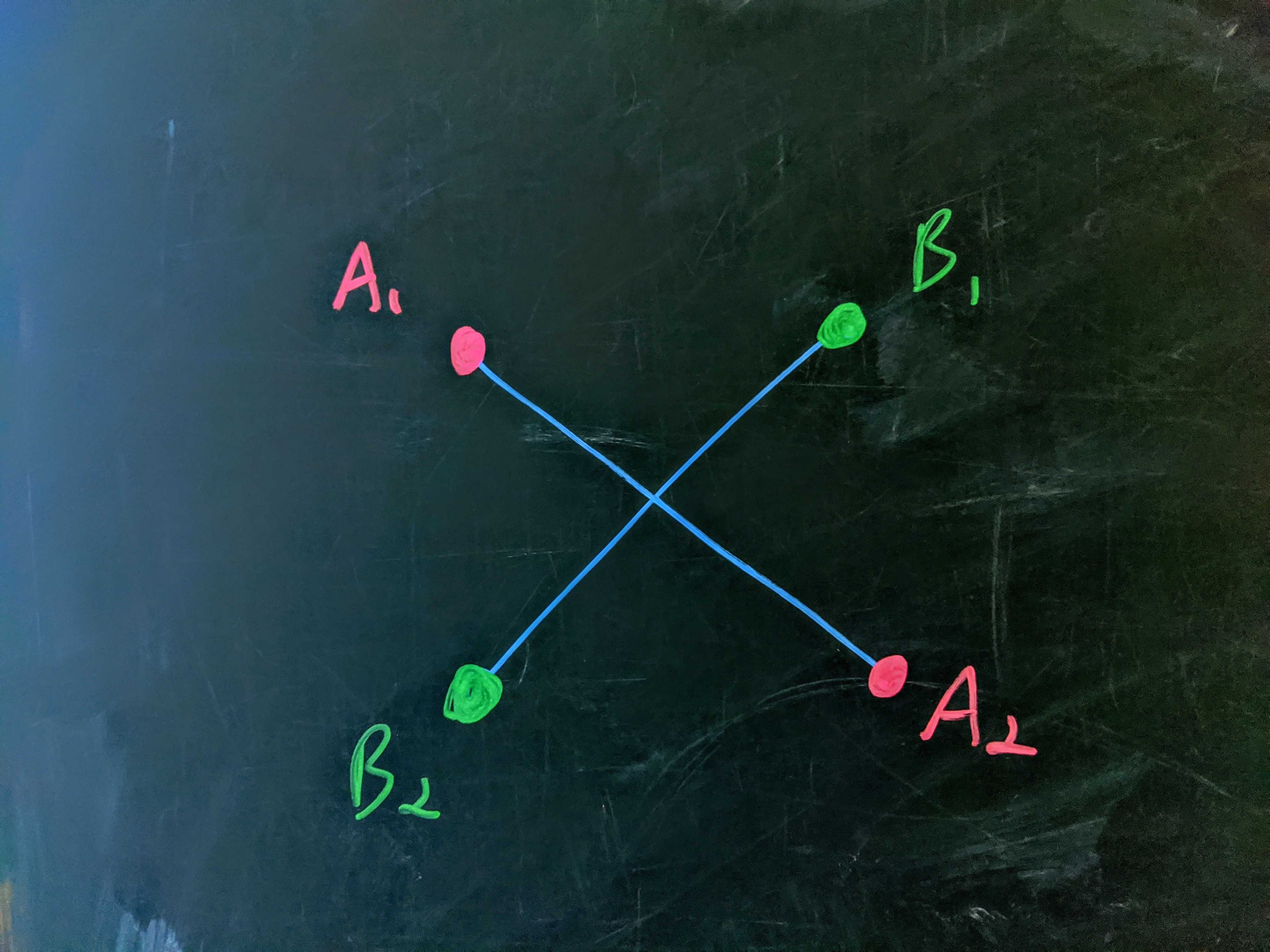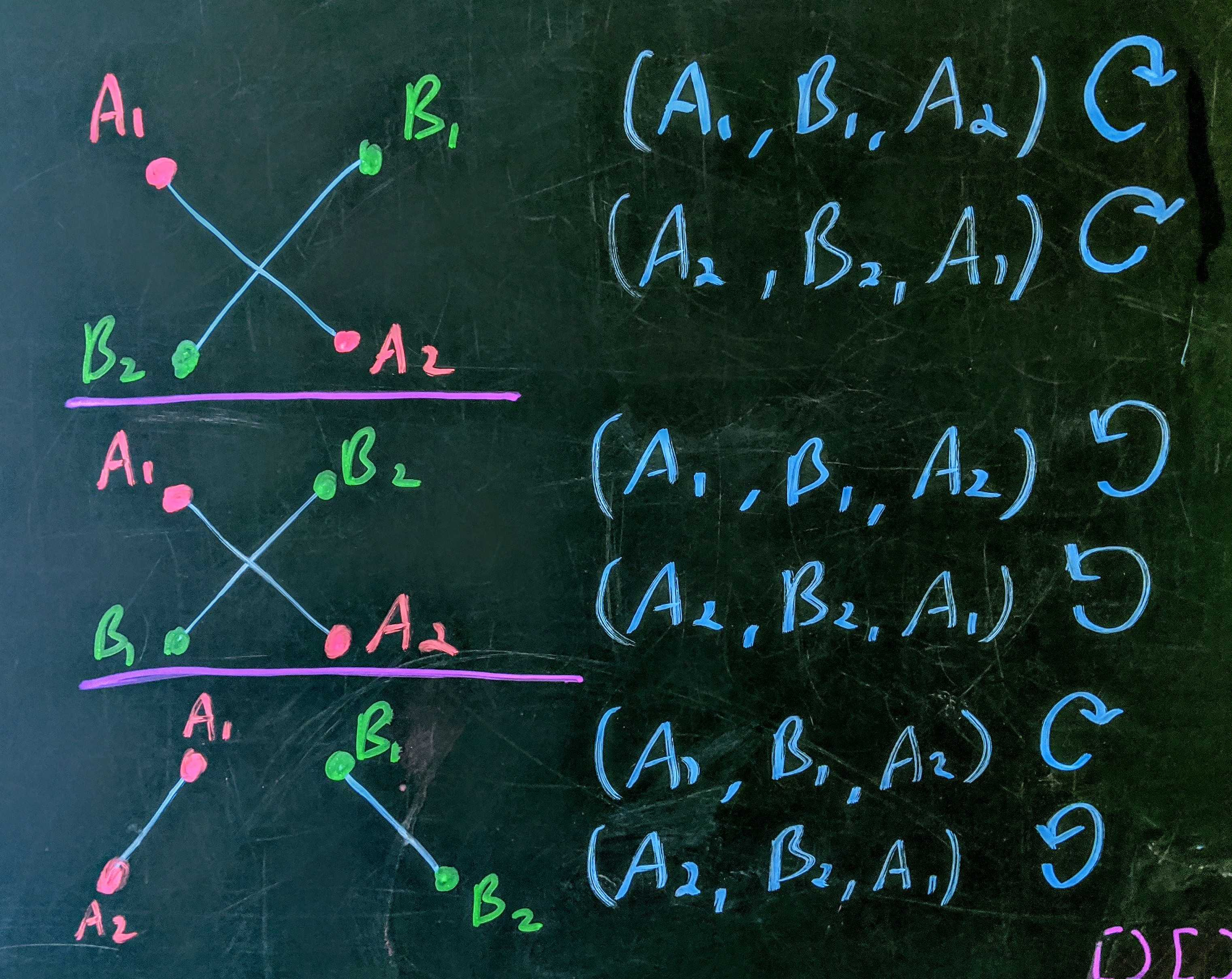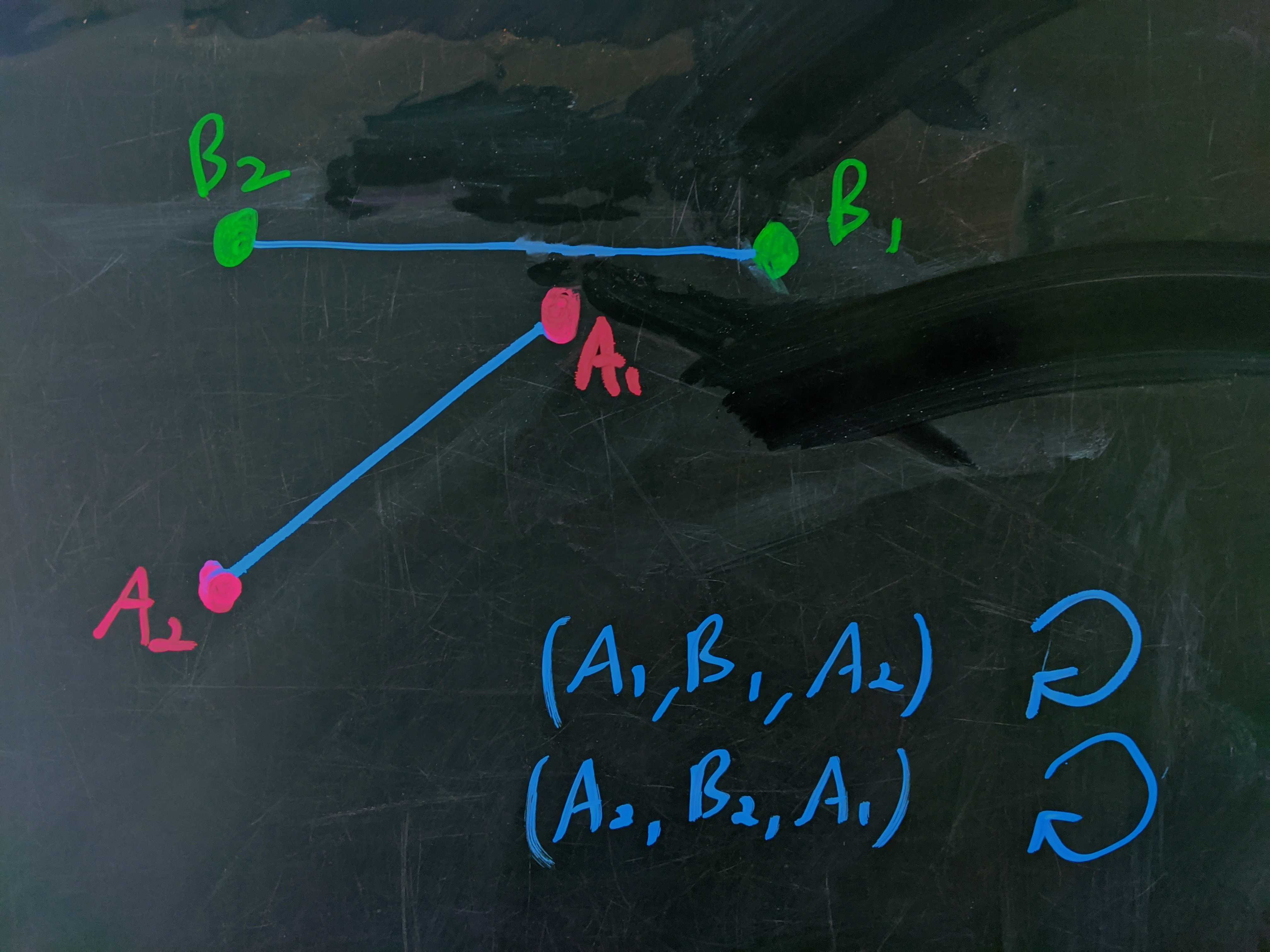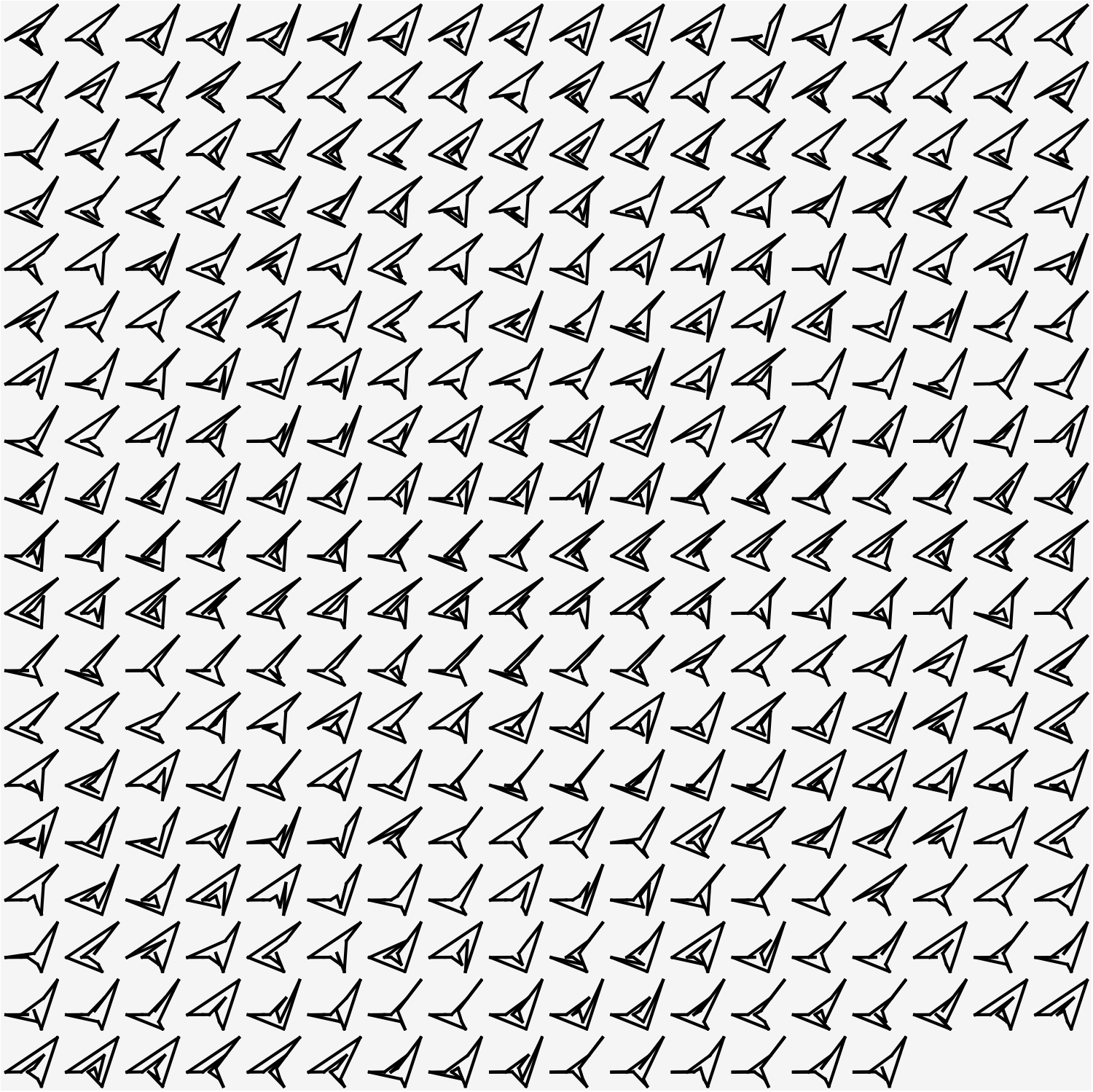Question: Suppose you have a pencil, a hexagon, and absolutely nothing to do. What is the greatest number of knockoff hexagons you can draw using the same $6$ points as the original hexagons? What about septagons? What about octagons? What about …

## Solution

As I have no real insight into the geometry of the problem, my basic approach is to generate $N$ random points, form all possible $N$-gons from them, check them all for intersections, and move on to a new set of random points if the old maximum isn’t deposed. The trick is being able to tell when two lines have an intersection. The other idea is to fail quickly.

If we draw two lines on a page, it’s easy to come up with a condition for the crossing.Crossing arrangement.

\begin{align} A_1.x &< B_1.x \\ A_1.y &> B_2.y \\ B_2.x &< A_2.y \\ B_1.y &> A_2.y \end{align}

But this is too naive since, when we generate the points randomly, we won’t know what order the points will be in. With a little bit of care we can make it robust to order.

It’s always going to be the case that if we take two points from one of the lines and one point from the other, whichever way we do this, they’ll have to have the same orientation, $\mathcal{O}$ (clockwise or counterclockwise).Orientation conditions for two crossings, and a non-crossing.

So, we can check if the orientations $\mathcal{O}(A_1,B_1,A_2)$ and $\mathcal{O}(A_2,B_2,A_1)$ match (both clockwise or both counterclockwise). That’s fine if the edges have the same length. But, if one is longer than the other, we can satisfy this without the lines actually crossing. To take care of that possibility we just have to do the same check for two points of line B and one point from line A.Counter example for simple two-corner conditions.

Our final condition for intersection is:

$\mathcal{O}(A_1,B_1,A_2) = \mathcal{O}(A_2,B_2,A_1)\ \mathbf{AND}\ \mathcal{O}(B_1,A_1,B_2) = \mathcal{O}(B_2,A_2,B_1)$

intersection[lineA_, lineB_] := (
If[Length@DeleteDuplicates@Flatten[{lineA, lineB}, 1] != 4, Return[False]];
If[orientation[lineA[], lineA[], lineB[]] !=
orientation[lineA[], lineA[], lineB[]], Return[False]];
If[orientation[lineB[], lineB[], lineA[]] !=
orientation[lineB[], lineB[], lineA[]], Return[False]];
Return[True];
)


With that in place, we need a function that can determine the orientation of three points ($+1$ for counterclockwise, $-1$ for clockwise):


ccw[ptA_, ptB_, ptC_] := (
Return[(ptB[] - ptA[]) (ptC[] - ptA[]) - (ptC[] -
ptA[]) (ptB[] - ptA[])]
)


The next thing we need is a map from a set of values $\{v_1,\ldots,v_N\}$ to the set of unique permutations of those values. To do this, I start with a set of symbols that I pair off into points ($(a,b), (c,d), \ldots$) and then permute into all possible orders. I then pair this list of points off to form lines. Finally, since this process creates redundancies, I delete the duplicates. This produces a symbolic list of all possible point orders that can then be fed specific values for the coordinates $(a,b,c,\ldots)$ to produce all potential $n$-gons.:

alph = {a, b, c, d, e, f, g, h, i, j, k, l, m, n, o, p, q, r,
s, t, u, v, w, x, y, z}

pointsMap[NN_] := (
points = {alph[[2 # - 1]], alph[[2 #]]} & /@ Range[NN];

(* add the first point at the end, to cut down on redundancy
prior to deduping *)
perms = Permutations[points[[2 ;; -1]]];
perms = Join[{points[]}, #] & /@ perms;

(* turn each permutation into a set of edges *)
setsOfLines =
Table[{#[[i]], #[[1 + i~Mod~NN]]}, {i, 1, NN}] & /@ perms;

(* sort the lines within each edge set, and
then sort the edge set, then deduplicate *)
tmp = SortBy[#, First] & /@ # & /@ setsOfLines;
tmp = Sort /@ tmp;
tmp = DeleteDuplicates[tmp];
Return[tmp];
)


From here, the approach is simply to hill climb. The logic is

1. Generate a set of random values for the coordinates.
2. Go through all its possible N-gons and count how many had intersections.
3. If that's lower than the current minimum, set it as the new minimum.
4. Repeat.


This is a probabilistic approach and, so, you can never know that you’ve actually found an optimal set. But you can put a lower bound on the probability of any potential optima that are still hiding. In the code below, we bail out of a set of random points as soon as we determine that it can’t beat the current optimum.

hasNoIntersection[setOfLines_] :=
(
(* loop over all ordered pairs of edges looking for an intersection.
if there isn't one, return True. *)
pairs = Subsets[setOfLines, {2}];
For[i = 1, i <= Length@pairs, i++,
If[intersection[pairs[[i]][], pairs[[i]][]], Return[False]];
];
Return[True]
)

NN = 6
(* make the map once, before the loop *)
maps = pointsMap[NN];

tryRandomSet[] :=
(
(* generate random coordinate values for the points *)
data = maps /. Table[alph[[i]] -> RandomReal[], {i, 1, 2*NN}];
intersectionCount = 0;

(* go through all edge sets, checking for intersections,
if there's no intersection, increase the count of intersection-free
N-gons. if there are already too many invalid N-gons for this to be
a new optimum, move on to the next round. *)
For[j = 1, j <= Length@data, j++,
If[intersectionCount >= minCount - 1, Return[Infinity]];
If[! hasNoIntersection[data[[j]]], intersectionCount++];
];
minCount = intersectionCount;
Print[Length@data - intersectionCount];
AppendTo[pointsRecord, data];
)

minCount = Infinity;
pointsRecord = {};

Do[
tryRandomSet[];
If[round~Mod~100 == 0, Print["Round: " <> ToString[round]];
Print[minCount]];,
{round, 1, 10000}
];


For $N \leq 7$, the algorithm runs for a few seconds before stalling indefinitely, presumably at the maximum. The $N=8$ run continued hill climbing for about $40\text{ min}$ before finding an arrangement with $339$ different octagons after which it found no better arrangement for $5\text{ hours}.$

$\begin{array}{c|c} N & \text{Unique N-gons} \\ \hline 3 & 1 \\ 4 & 3 \\ 5 & 8 \\ 6 & 29 \\ 7 & 92 \\ 8 & 339 \end{array}$

Here we present, in all its majesty, $339$ unique octagons using one of the maximal octagonal sets the algorithm found:A set of $339$ unique octagons generated from the same $8$ points.

I tried going for a more involved genetic approach, being less aggressive in repicking random points as the score improves, but I ran out of Saturday.